Methods and formulas for Double Exponential Smoothing

Select the method or formula of your choice.

Model equation

Double exponential smoothing employs a level component and a trend component at each period. Double exponential smoothing uses two weights, (also called smoothing parameters), to update the components at each period. The double exponential smoothing equations are as follows:

Formula

Lt = α Yt + (1 – α) [Lt –1 + Tt –1]

Tt = γ [Lt Lt –1] + (1 – γ) Tt –1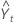= Lt –1 + Tt –1

If the first observation is numbered one, then level and trend estimates at time zero must be initialized in order to proceed. The initialization method used to determine how the smoothed values are obtained in one of two ways: with optimal weights or with specified weights.

Notation

TermDescription
Lt level at time t
α weight for the level
Tt trend at time t
γ weight for the trend
Yt data value at time tfitted value, or one-step-ahead forecast, at time t

Weights

Optimal ARIMA weights

1. Minitab fits with an ARIMA (0,2,2) model to the data, in order to minimize the sum of squared errors.
2. The trend and level components are then initialized by backcasting.

Specified weights

1. Minitab fits a linear regression model to time series data (y variable) versus time (x variable).
2. The constant from this regression is the initial estimate of the level component, the slope coefficient is the initial estimate of the trend component.

When you specify weights that correspond to an equal-root ARIMA (0, 2, 2) model, Holt's method specializes to Brown's method1.

Forecasts

Double exponential smoothing uses the level and trend components to generate forecasts. The forecast for m periods ahead from a point at time t is as follows:

Formula

Lt + mTt

Data up to the forecast origin time are used for the smoothing.

Notation

TermDescription
Lt level at time t
Tt trend at time t

Prediction limits

Formula

Based on the mean absolute deviation (MAD). The formulas for the upper and lower limits are as follows:
• Upper limit = Forecast + 1.96 × dt × MAD
• Lower limit = Forecast – 1.96 × dt × MAD

Notation

TermDescription
β max{α, γ)
δ 1 – β
α level smoothing constant
γ trend smoothing constant
τ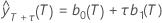b 0(T)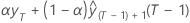b 1(T)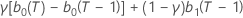MAPE

Mean absolute percentage error (MAPE) measures the accuracy of fitted time series values. MAPE expresses accuracy as a percentage.

Notation

TermDescription
yt actual value at time t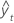fitted value
n number of observations

Mean absolute deviation (MAD) measures the accuracy of fitted time series values. MAD expresses accuracy in the same units as the data, which helps conceptualize the amount of error.

Notation

TermDescription
yt actual value at time tfitted value
n number of observations

MSD

Mean squared deviation (MSD) is always computed using the same denominator, n, regardless of the model. MSD is a more sensitive measure of an unusually large forecast error than MAD.

Notation

TermDescription
yt actual value at time tfitted value
n number of observations
1 N.R. Farnum and L.W. Stanton (1989). Quantitative Forecasting Methods. PWS-Kent.
By using this site you agree to the use of cookies for analytics and personalized content.  Read our policy